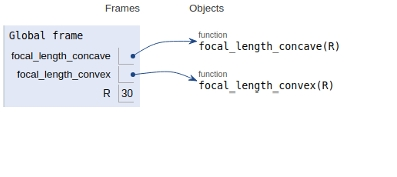# The focal length of a spherical mirror in Python Program

PythonServer Side ProgrammingProgramming

## Problem statement

We will be given the radius of curvature of the spherical mirror and we have to find the focal length of the same.

The focal length is the distance between the centre of curvature of the mirror to the principal foci . In order to determine the focal length of a spherical mirror firstly, we should know the radius of curvature of that mirror. The distance from the vertex of the mirror to the centre of curvature is called the radius of curvature.

Mathematically −

For concave mirror: F = R/2

For convex mirror: F = -R/2

Now let’s see the implementation

## Example

Live Demo

#spherical concave mirror
def focal_length_concave(R):
return R / 2
# spherical convex mirror
def focal_length_convex(R):
return - ( R/ 2 )
# Driver function
R = 30
print("Focal length of spherical concave mirror is :", focal_length_concave(R)," units")
print("Focal length of spherical convex mirror is : ", focal_length_convex(R)," units")

## Output

Focal length of spherical concave mirror is: 15.0 units
Focal length of spherical convex mirror is: -15.0 units

The Outputs can be in metre, centimetre or millimetre. For generalized result units is mentioned in place of any specific unit type.

All the variables are declared in the global frame with two functions as shown in the image below.## Conclusion

In this article, we learnt about how we can calculate the focal length of a spherical mirror.